If the resistance of voltmeter is 10000Ω and resistance of ammeter is 2Ω then find R when voltmeter reads 12V and ammeter reads 0.1 A :

(1) 118 Ω

(2) 120 Ω

(3) 124 Ω

(4) 114Ω

Concept Questions :-

Kirchoff's voltage law
High Yielding Test Series + Question Bank - NEET 2020

Difficulty Level:

Potentiometer wire of length 1 m is connected in series with 490 Ω resistance and 2V battery. If 0.2 mV/cm is the potential gradient, then the resistance of the potentiometer wire is :

(1) 4.9 Ω

(2) 7.9 Ω

(3) 5.9 Ω

(4) 6.9 Ω

Concept Questions :-

Meter bridge and potentiometer
High Yielding Test Series + Question Bank - NEET 2020

Difficulty Level:

In an electrical cable, there is a single wire of radius 9 mm of copper. Its resistance is 5 Ω. The cable is replaced by 6 different insulated copper wires, the radius of each wire is 3 mm. Now the total resistance of the cable will be :

(1) 7.5 Ω

(2) 45 Ω

(3) 90 Ω

(4) 270 Ω

Concept Questions :-

Combination of resistors
High Yielding Test Series + Question Bank - NEET 2020

Difficulty Level:

Two uniform wires A and B are of the same metal and have equal masses. The radius of wire A is twice that of wire B. The total resistance of A and B when connected in parallel is :

(1) 4 Ω when the resistance of wire A is 4.25 Ω

(2) 5 Ω when the resistance of wire A is 4.25 Ω

(3) 4 Ω when the resistance of wire B is 4.25 Ω

(4) 4 Ω when the resistance of wire B is 4.25 Ω

Concept Questions :-

Derivation of Ohm's law
High Yielding Test Series + Question Bank - NEET 2020

Difficulty Level:

Twelve wires of equal length and same cross-section are connected in the form of a cube. If the resistance of each of the wires is R, then the effective resistance between the two diagonal ends would be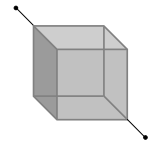(1) 2R

(2) 12R

(3) $\frac{5}{6}R$

(4) 8R

Concept Questions :-

Combination of resistors
High Yielding Test Series + Question Bank - NEET 2020

Difficulty Level:

You are given several identical resistances each of value R = 10 Ω and each capable of carrying maximum current of 1 ampere. It is required to make a suitable combination of these resistances to produce a resistance of 5 Ω which can carry a current of 4 amperes. The minimum number of resistances of the type R that will be required for this job

(1) 4

(2) 10

(3) 8

(4) 20

Concept Questions :-

Combination of resistors
High Yielding Test Series + Question Bank - NEET 2020

Difficulty Level:

The resistance of a wire is 10–6 Ω per metre. It is bend in the form of a circle of diameter 2 m. A wire of the same material is connected across its diameter. The total resistance across its diameter AB will be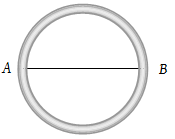(1) $\frac{4}{3}\pi ×{10}^{-6}\Omega$

(2) $\frac{2}{3}\pi ×{10}^{-6}\Omega$

(3) $0.88×{10}^{-6}\Omega$

(4) $14\pi ×{10}^{-6}\Omega$

Concept Questions :-

Combination of resistors
High Yielding Test Series + Question Bank - NEET 2020

Difficulty Level:

In the figure shown, the capacity of the condenser C is $2\text{\hspace{0.17em}}\mu F$. The current in 2 Ω resistor is :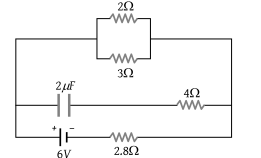(1) 9 A

(2) 0.9 A

(3) $\frac{1}{9}A$

(4) $\frac{1}{0.9}A$

Concept Questions :-

Kirchoff's voltage law
High Yielding Test Series + Question Bank - NEET 2020

Difficulty Level:

When the key K is pressed at time t = 0, which of the following statements about the current I in the resistor AB of the given circuit is true?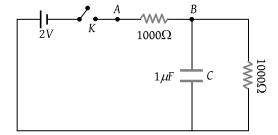(1) I = 2 mA at all t

(2) I oscillate between 1 mA and 2mA

(3) I = 1 mA at all t

(4) At t = 0 , I = 2 mA and with time it goes to 1 mA

Concept Questions :-

Kirchoff's voltage law
High Yielding Test Series + Question Bank - NEET 2020

Difficulty Level:

A torch bulb rated as 4.5 W, 1.5 V is connected as shown in the figure. The e.m.f. of the cell needed to make the bulb glow at full intensity is :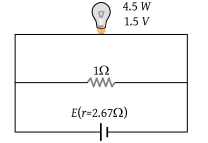(1) 4.5 V

(2) 1.5 V

(3) 2.67 V

(4) 13.5 V

Concept Questions :-

Heating effect of current